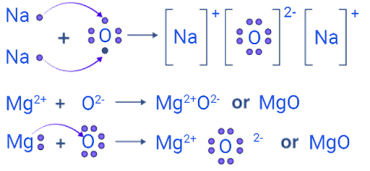# Class 10 Science | Intext Questions & Answers | Chapter 3 | Metals and Non-metals

Page: 40

1.   Give an example of a metal which:

(i)      is a liquid at room temperature.

(ii)     can be easily cut with a knife.

(iii)    is the best conductor of heat.

(iv)    is a poor conductor of heat.

(i)      Mercury                       (ii)  Sodium

2.   Explain the meanings of malleable and ductile.

Malleable means a metal can be beaten into thin sheets.

Ductile means a metal can be drawn into thin wires.

Page: 46

1.   Why is sodium kept immersed in kerosene oil?

Sodium is highly reactive. So it is kept immersed in kerosene oil to prevent reaction with atmospheric oxygen, moisture and CO2.

2.   Write equations for the reactions of

(i)    iron with steam.

(ii)   calcium and potassium with water.

(i)    3Fe (s) + 4H2O (g) Fe3O4 (s) + 4H2 (g)

(ii)   Ca(s) + 2H2O(l) Ca(OH)2(aq)  +  H2(g)

2K(s) + 2H2O(l) 2KOH(aq)  +  H2(g) + heat

3.   Samples of four metals A, B, C and D were taken and added to the following solution one by one.

The results obtained have been tabulated as follows:

 Metal Iron (II) sulphate Copper (II) sulphate Zinc sulphate Silver nitrate A No reaction Displacement B Displacement No reaction C No reaction No reaction No reaction Displacement D No reaction No reaction No reaction No reaction

Use the Table above to answer the following questions about metals A, B, C and D.

(i)  Which is the most reactive metal?

(ii) What would you observe if B is added to a solution of copper (II) sulphate?

(iii)Arrange the metals A, B, C and D in the order of decreasing reactivity.

(i) B is the most reactive metal because it gives displacement reaction with iron (II) sulphate.

(ii) When metal B is added to copper (II) sulphate solution, a displacement reaction will take place due to which the blue colour of copper (II) sulphate solution will fade and a red-brown deposit of copper will be formed on metal B.

(iii)  B > A > C > D.

4.  Which gas is produced when dilute hydrochloric acid is added to a reactive metal? Write the chemical reaction when iron reacts with dilute H2SO4.

Hydrogen gas is produced.

Chemical reaction between iron and dilute H2SO4:

Fe(s) + H2SO4(aq) FeSO4(aq) + H2(g)

5. What would you observe when zinc is added to a solution of iron (II) sulphate? Write the chemical reaction that takes place.

Zinc is more reactive than iron. Therefore, when zinc is added to iron (II) sulphate solution, the greenish colour of the solution fades gradually due to the formation of colourless zinc sulphate solution and iron metal is deposited on zinc.

FeSO4(aq) + Zn(s) + ZnSO4(aq) + Fe(s)

Page: 49

1. (i) Write the electron dot structures for sodium, oxygen and magnesium.

(ii) Show the formation of Na2O and MgO by the transfer of electrons.

(iii) What are the ions present in these compounds?

 (i) l Na ll l O l ll ll Mg (ii) Formation of Na2O and MgO:(iii) Ions present in Na2O: Na+ and O2-. Ions present in In MgO: Mg2+ and O2-.

2.  Why do ionic compounds have high melting points?

In ionic compounds, there is a strong force of attraction between the oppositely charged ions. So, much heat energy is needed to break this force of attraction and melt the ionic compound. Therefore, ionic compounds have high melting points.

Page: 53

1.   Define the following terms: (i) Mineral, (ii) Ore and (iii) Gangue.

(i)    Mineral: These are the natural materials in which the metals or their compounds are found.

(ii)   Ore: These are the minerals from which the metals can be extracted conveniently and profitably.

(iii)  Gangue: These are the unwanted impurities like sand, rocky material, earth particles, lime stone, etc. in an ore.

2.   Name two metals which are found in nature in the free state.

Gold and platinum

3.   What chemical process is used for obtaining a metal from its oxide?

Reduction.

E.g., zinc oxide is reduced to metallic zinc by heating with carbon.

ZnO(s) + C(s) Zn(s) + CO(g)

4.   Gold is Metal or Nonmetal?

Gold is a metal found in nature in the free state

Page: 55

1.   Metallic oxides of zinc, magnesium and copper were heated with the following metals:

 Metal Zinc Magnesium Copper 1 Zinc oxide 2 Magnesium oxide 3 Copper oxide

In which cases will you find displacement reactions taking place?

A more reactive metal can displace a less reactive metal from its oxide. Here, magnesium is most reactive, zinc is less reactive and copper is least reactive.

 Metal Zinc Magnesium Copper 1 Zinc oxide – Displacement – 2 Magnesium oxide – – – 3 Copper oxide Displacement Displacement –

2.   Which metals do not corrode easily?

Gold and Platinum.

3.   What are alloys?